# Background/Purpose¶

A collapsible sidebar was deployed as an opt-out feature to test wikis as part of the new vector skin on desktop. This was the first change as part of the Desktop Improvements Project and allows users to collapse the lengthy menu found on the left side of each page.

The sidebar was deployed as open by default for all users and the status preserved for logged-in users across sessions. The purpose of this analysis is to investigate how the collapsible sidebar is affecting user behavior.

Data includes events recorded from 22 July 2020 through 31 August 2020 from the DesktopWebUIActionsSchema. We also reviewed events recorded in pageview_hourly table and webrequest to understand dekstop interaction prior to the deployment of the collapsible sidebar and instrumentation.

Deployment Dates

project Wed. July 22 Tues. July 28 Wed. Aug 5
euwiki (Basque Wikipedia) x
fawiki (Persian Wikipedia) x
frwiki (French Wikipedia) x
frwiktionary (French Wiktionary) x
hewiki (Hebrew Wikipedia) x
ptwikiversity (Portuguese Wikiversity) x
In :
library(IRdisplay)

display_html(
'<script>
code_show=true;
function code_toggle() {
if (code_show){
$(\'div.input\').hide(); } else {$(\'div.input\').show();
}
code_show = !code_show
}
$( document ).ready(code_toggle); </script> <form action="javascript:code_toggle()"> <input type="submit" value="Click here to toggle on/off the raw code."> </form>' )  In : shhh <- function(expr) suppressPackageStartupMessages(suppressWarnings(suppressMessages(expr))) shhh({ library(tidyverse); library(glue); library(lubridate); library(scales) })  In : options(repr.plot.width = 15, repr.plot.height = 7)  In : #deployment annotations to use in charts vertical_lines <- as.numeric(as.Date(c("2020-07-22", "2020-07-28", "2020-08-05")))  # What is the frequency that users collapse and uncollapse the sidebar?¶ ## Approach¶ Reviewed the following: • Number of clicks to the sidebar and average clicks per session overall and by wiki, edit count, and logged in status. • Percent of sessions with clicks to the sidebar (either to collapse or to uncollapse) • Frequency of number of clicks to the sidebar per session Note: In the section below, a sidebar click is defined as a click to either collapse or uncollapse the sidebar. ## What are the total and average clicks to the sidebar per session?¶ In : #collect sidebar clicks by edit count, sidebar state and wiki query <- "SELECT date_format(dt, 'yyyy-MM-dd') AS date, event.token AS session, wiki AS wiki, event.isAnon AS logged_in_status, event.isSidebarCollapsed AS sidebar_state, event.editCountBucket AS user_edit_count, COUNT(*) as events FROM event.desktopwebuiactionstracking WHERE -- review clicks to the sidebar event.name = 'ui.sidebar' AND event.action = 'click' AND year = 2020 AND ((month=07 AND day >= 22) OR month= 08 ) -- sidebar is collapsible only on new vector skin AND event.skinversion = 2 AND wiki <> 'testwiki' AND useragent.is_bot = false GROUP BY date_format(dt, 'yyyy-MM-dd'), event.token, event.isAnon, event.isSidebarCollapsed, wiki, event.editCountBucket "  In : sidebar_clicks <- wmfdata::query_hive(query)  Don't forget to authenticate with Kerberos using kinit  In : sidebar_clicks$date <- as.Date(sidebar_clicks$date, format = "%Y-%m-%d")  In : sidebar_clicks$sidebar_state <- ifelse(sidebar_clicks$sidebar_state == 'false', "uncollapse", "collapse")  In : sidebar_clicks$logged_in_status <- ifelse(sidebar_clicks\$logged_in_status == 'false', "logged-in", "logged-out")


### Overall¶

In :
# Number of collapse events vs uncollapse events

p <- sidebar_clicks %>%
group_by(date, sidebar_state) %>%
summarise(total_events = sum(events)) %>%
ggplot(aes(x=date, y= total_events, color = sidebar_state)) +
geom_line(size = 1.5) +
geom_vline(xintercept = vertical_lines,
linetype = "dashed", color = "black") +
geom_text(aes(x=as.Date('2020-07-22'), y=1E3, label="New skin deployed on Basque Wiki, Fr wiktionary, Pt wikiversity"), size=3.7, vjust = -1.2, angle = 90, color = "black") +
geom_text(aes(x=as.Date('2020-07-28'), y=1E3, label="New skin deployed on Persian and Hebrew Wikipedias"), size=3.7, vjust = -1.2, angle = 90, color = "black") +
geom_text(aes(x=as.Date('2020-08-05'), y=1E3, label="New skin deployed on French Wikipedia"), size=3.7, vjust = -1.2, angle = 90, color = "black") +
scale_x_date("Date", labels = date_format("%d %b %Y"), date_breaks = "1 week") +
scale_y_continuous("Number of clicks per day") +
labs (title = "Daily sidebar clicks by sidebar state")  +
theme_bw() +
theme(
plot.title = element_text(hjust = 0.5),
axis.text.x = element_text(angle = 45, vjust = 0.5, hjust=0.5),
text = element_text(size=18))
p

ggsave("Figures/daily_sidebar_clicks_overall.png", p, width = 16, height = 8, units = "in", dpi = 300)

summarise() regrouping output by 'date' (override with .groups argument)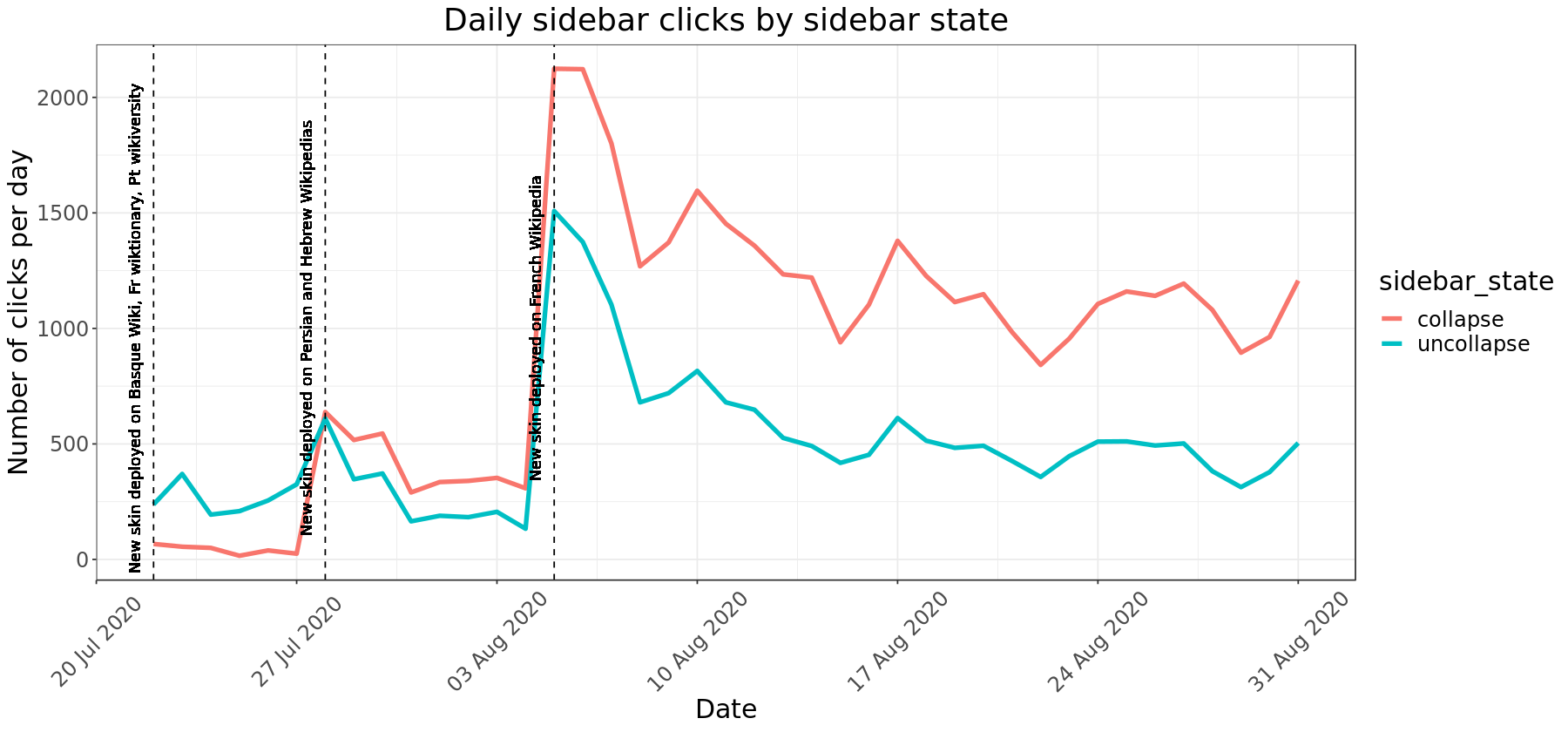#### Overall Avearge Clicks Per Session¶

In :
count_events_overall <- sidebar_clicks %>%
summarize(num_events = sum(events),
num_sessions = n_distinct(session),
avg_clicks = num_events/num_sessions)

count_events_overall

A data.frame: 1 × 3
num_eventsnum_sessionsavg_clicks
<int><int><dbl>
57703287232.008948

#### Overall Avearge Clicks Per Session By Sidebar State¶

In :
count_events_bysidebarstatus <- sidebar_clicks %>%
group_by(sidebar_state) %>%
summarize(num_events = sum(events),
num_sessions = n_distinct(session),
avg_clicks = num_events/num_sessions)

count_events_bysidebarstatus

summarise() ungrouping output (override with .groups argument)


A tibble: 2 × 4
sidebar_statenum_eventsnum_sessionsavg_clicks
<chr><int><int><dbl>
collapse 37566275021.365937
uncollapse20137146351.375948

There is a sharp increases in number of clicks to the sidebar (both to either collapse or uncollapse) following each deployment. Following the last deployment to French Wikipedia on August 5th, the number of clicks to the sidebar have begun to stabilize to around 1400 to 1700 clicks per day.

From the first deployment on July 22nd to the end of August 2020, there have been a total of 57,703 clicks to either collapse or uncollapse sidebar by 28,723 unique search sessions. There is an average 2 sidebar clicks per session.

Since the sidebar was set as uncollapsed by default, there are more clicks to collapse the sidebar. There are roughly the same average number of clicks per session (1.3) to either collapse or uncollapse.

### By Wiki¶

In :
#Chart overall sidebar clicks over time by wiki

p <- sidebar_clicks %>%
group_by(date, wiki) %>%
summarise(total_clicks = sum(events),
avg_clicks = sum(events)/n_distinct(session)) %>%
ggplot(aes(x=date, y= total_clicks, color = wiki)) +
geom_line(size = 1.5) +
geom_vline(xintercept = vertical_lines,
linetype = "dashed", color = "black") +
geom_text(aes(x=as.Date('2020-07-22'), y=2.5, label="New skin deployed on Basque Wiki, French wiktionary, Portuguese wikiversity"), size=3.7, vjust = -1.2, angle = 90, color = "black") +
geom_text(aes(x=as.Date('2020-07-28'), y=2.5, label="New skin deployed on Persian and Hebrew Wikipedias"), size=3.7, vjust = -1.2, angle = 90, color = "black") +
geom_text(aes(x=as.Date('2020-08-05'), y=2.5, label="New skin deployed on French Wikipedia"), size=3.7, vjust = -1.2, angle = 90, color = "black") +
scale_x_date("Date", labels = date_format("%b %Y"), date_breaks = "1 week") +
scale_y_continuous("Number of clicks per day") +
labs (title = "Daily sidebar clicks by wiki")  +
theme_bw() +
theme(
plot.title = element_text(hjust = 0.5),,
text = element_text(size=18))
p

ggsave("Figures/daily_sidebar_clicks_bywiki.png", p, width = 16, height = 8, units = "in", dpi = 300)

summarise() regrouping output by 'date' (override with .groups argument)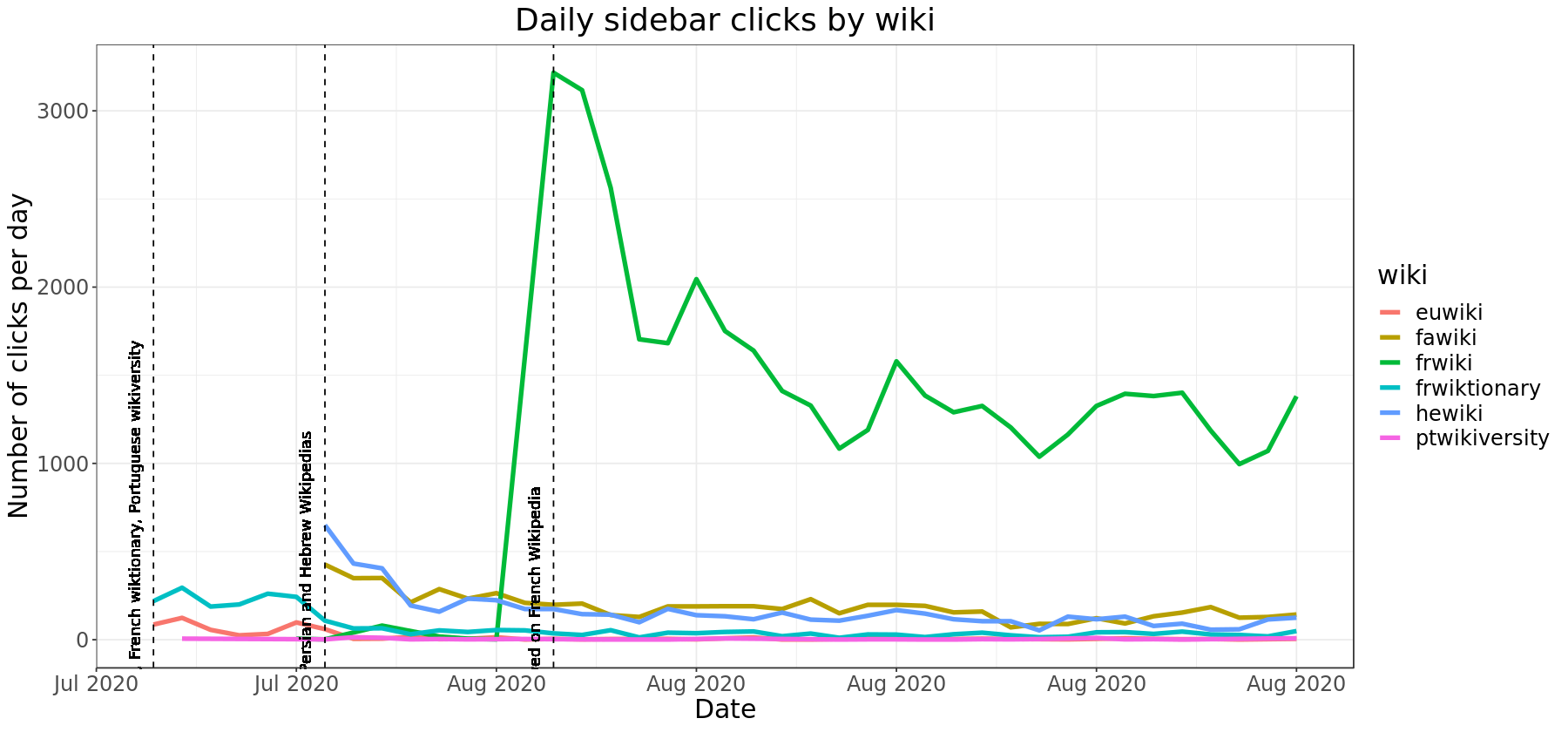#### Overall Average Clicks Per Session By Wiki¶

In :
# average clicks per wiki
sidebar_clicks_bywiki <- sidebar_clicks %>%
group_by(wiki) %>%
summarize(total_events = sum(events),
unique_sessions = n_distinct(session),
avg_events_persession = total_events/unique_sessions)

sidebar_clicks_bywiki

summarise() ungrouping output (override with .groups argument)


A tibble: 6 × 4
wikitotal_eventsunique_sessionsavg_events_persession
<chr><int><int><dbl>
euwiki 638 2602.453846
fawiki 6549 22232.946019
frwiki 41969219021.916218
frwiktionary 2733 14581.874486
hewiki 5704 28262.018401
ptwikiversity 110 542.037037

French Wiktionary had the lowest average sidebar clicks per session (1.87) while Persian Wikipedia had the highest (2.94)

In :
p <- sidebar_clicks %>%
group_by(date, wiki, sidebar_state) %>%
summarise(total_events = sum(events)) %>%
ggplot(aes(x = date, y= total_events, color = sidebar_state)) +
geom_line(size = 1.5) +
facet_wrap(~wiki, scales = "free") +
scale_x_date("Date", labels = date_format("%b %Y"), date_breaks = "1 week") +
scale_y_continuous("Number of clicks per day") +
labs (title = "Daily sidebar clicks by wiki and sidebar state")  +
theme_bw() +
theme(
plot.title = element_text(hjust = 0.5),,
text = element_text(size=18),
axis.text.x = element_text(angle = 45, hjust = 1))
p

ggsave("Figures/daily_sidebar_clicks_wiki_sidebarstate.png", p, width = 16, height = 8, units = "in", dpi = 300)

summarise() regrouping output by 'date', 'wiki' (override with .groups argument)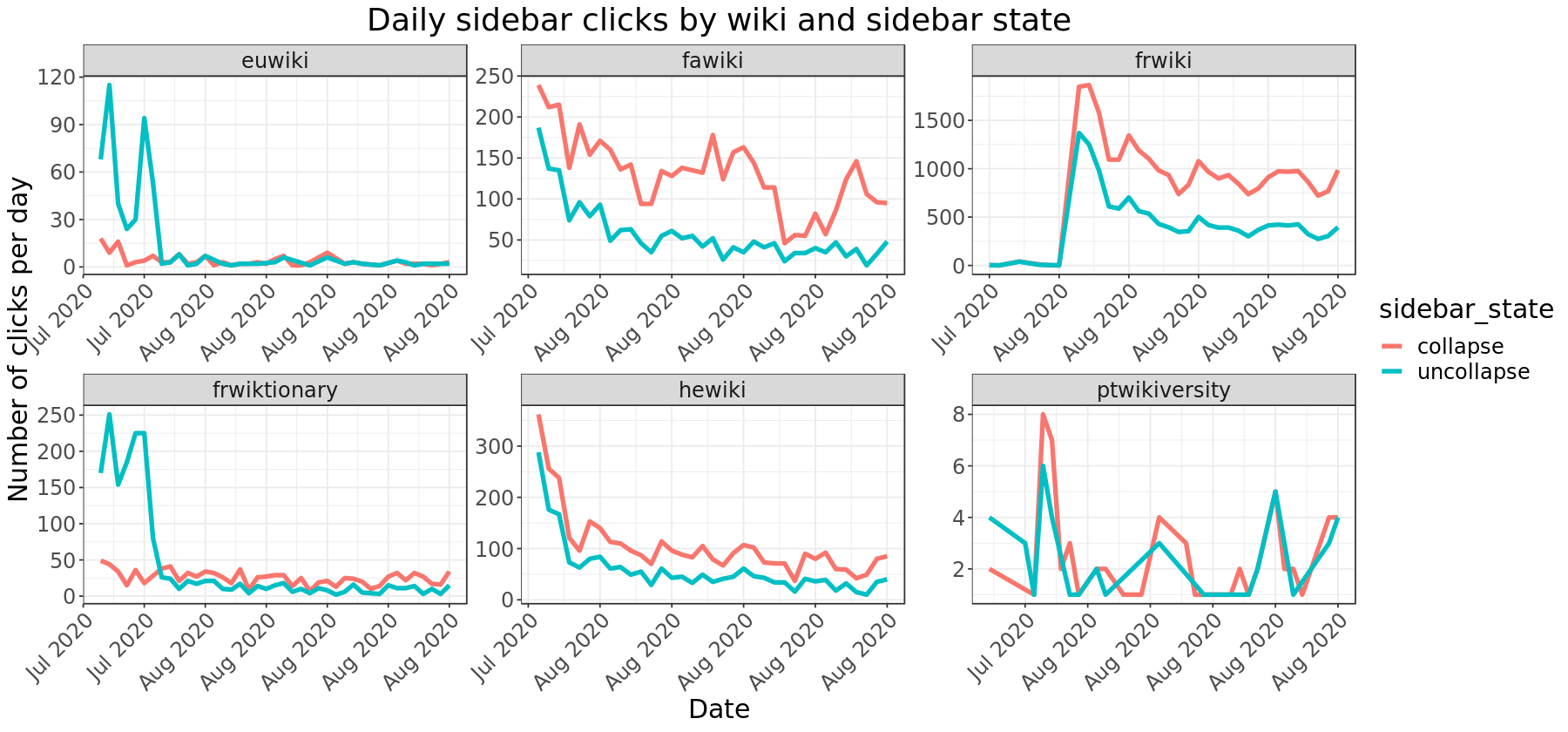In :
p <- sidebar_clicks %>%
group_by(wiki, sidebar_state) %>%
summarise(total_events = sum(events)) %>%
ggplot(aes(x = sidebar_state, y= total_events, fill = sidebar_state)) +
geom_bar(stat = 'identity') +
facet_wrap(~wiki, scales = "free") +
scale_y_continuous("Total number of clicks") +
labs (title = "Total sidebar clicks by wiki and sidebar state")  +
theme_bw() +
theme(
plot.title = element_text(hjust = 0.5),,
text = element_text(size=18),
axis.text.x = element_text(angle = 45, hjust = 1))
p

ggsave("Figures/total_sidebar_clicks_bywiki.png", p, width = 16, height = 8, units = "in", dpi = 300)

summarise() regrouping output by 'wiki' (override with .groups argument)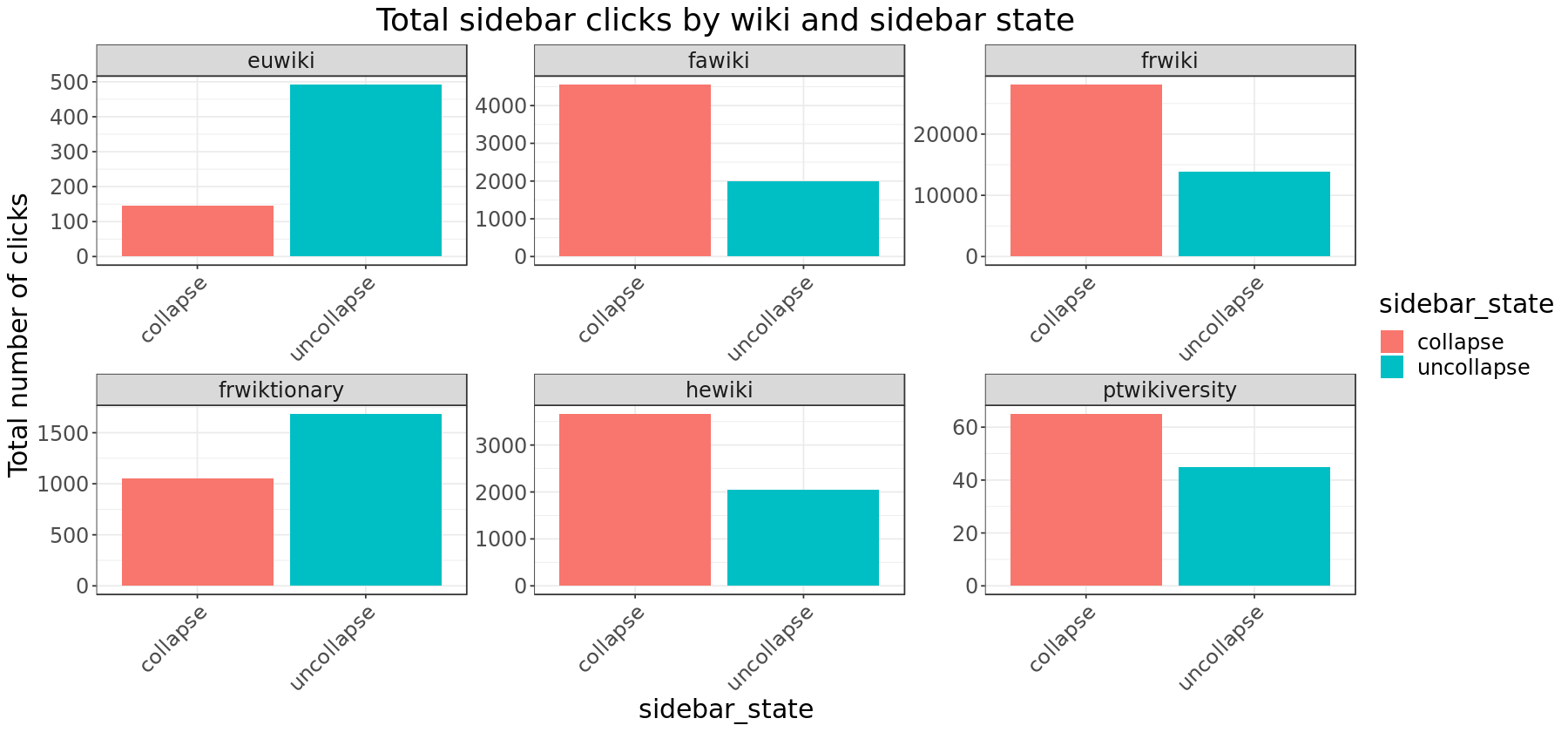There are a higher number of collapse events compared to uncollapse events for Persian Wikipedia, French Wikipedia, Hebrew Wikipedia and Portuguese Wikiversity, which makes sense as the sidebar was presented as uncollapsed as default. Ptwikiversity has a very low number of clicks to either collapse or uncollapse the sidebar (only 2 to 8 per day).

Interestingly, Basque Wikipedia and French Wiktionary had a higher number of clicks to uncollapse the sidebar despite the sidebar being uncollapsed as default. Both of these wikis also had a signficantly higher average number of uncollapse clicks per session.

#### Average Clicks Per Session By Wiki and Sidebar State¶

In :
# average clicks per wiki
sidebar_clicks_bywiki_sidebarstatus <- sidebar_clicks %>%
group_by(wiki, sidebar_state) %>%
summarize(total_events = sum(events),
unique_sessions = n_distinct(session),
avg_events_persession = total_events/unique_sessions)

sidebar_clicks_bywiki_sidebarstatus

summarise() regrouping output by 'wiki' (override with .groups argument)


A grouped_df: 12 × 5
wikisidebar_statetotal_eventsunique_sessionsavg_events_persession
<chr><chr><int><int><dbl>
euwiki collapse 146 1151.269565
euwiki uncollapse 492 2312.129870
fawiki collapse 4556 21692.100507
fawiki uncollapse 1993 13181.512140
frwiki collapse 28087216011.300264
frwiki uncollapse13882105451.316453
frwiktionary collapse 1049 8171.283966
frwiktionary uncollapse 1684 10521.600760
hewiki collapse 3663 27531.330548
hewiki uncollapse 2041 14571.400824
ptwikiversitycollapse 65 471.382979
ptwikiversityuncollapse 45 321.406250

### By Edit Count¶

In :
p <- sidebar_clicks %>%
# remove anonymous users
filter(logged_in_status == 'logged-in') %>%
group_by(date, user_edit_count) %>%
summarise(total_events = sum(events)) %>%
ggplot(aes(x=date, y= total_events, color = user_edit_count)) +
geom_line(size = 1.5) +
scale_y_continuous("Number of clicks per day") +
scale_x_date("Date", labels = date_format("%d %b %Y"), date_breaks = "1 week") +
labs (title = "Daily sidebar clicks by user edit count")  +
theme_bw() +
theme(
plot.title = element_text(hjust = 0.5),
text = element_text(size=18),
axis.text.x = element_text(angle = 45, hjust = 1))
p

ggsave("Figures/daily_sidebar_clicks_byusereditcount.png", p, width = 16, height = 8, units = "in", dpi = 300)

summarise() regrouping output by 'date' (override with .groups argument)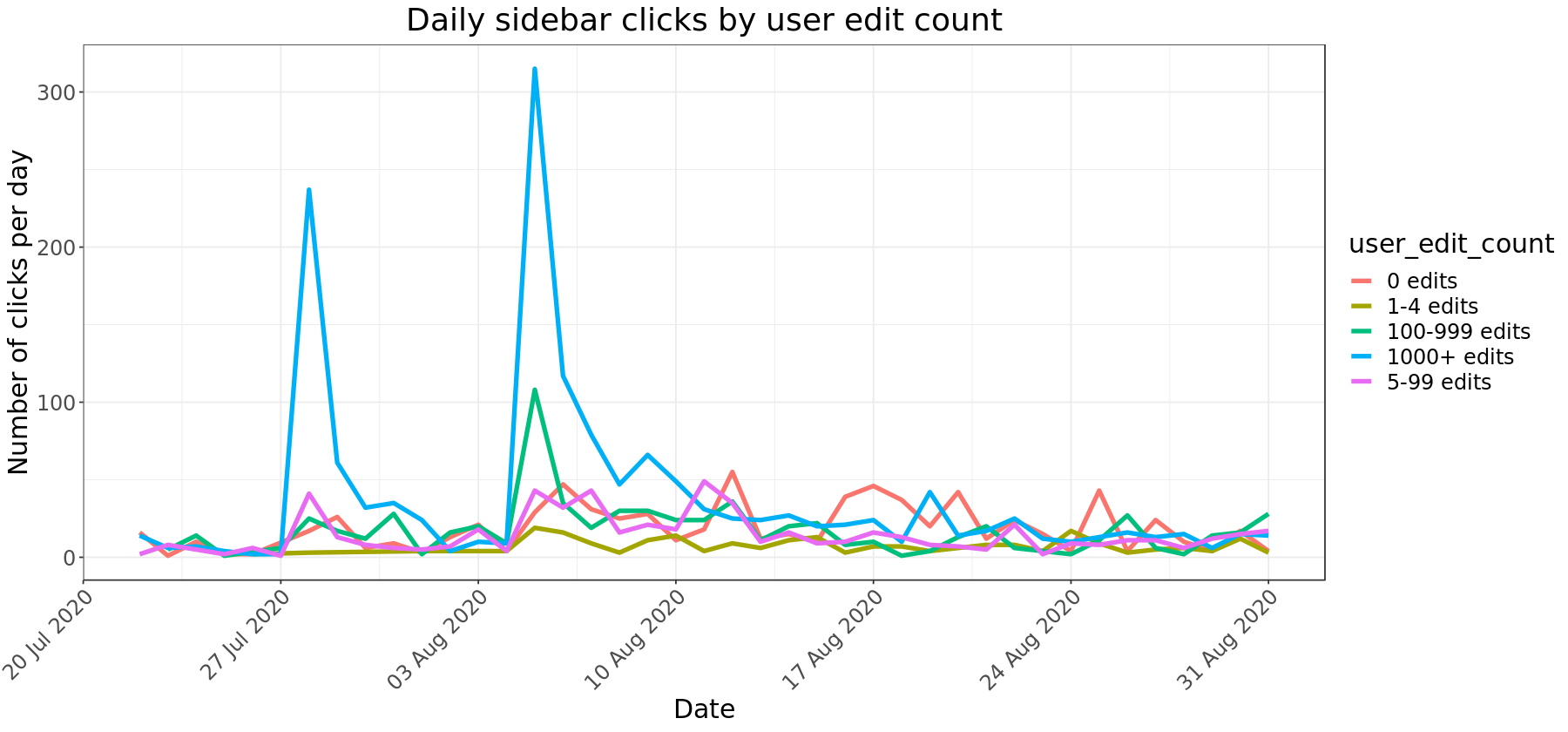#### Average sidebar clicks per session by edit count¶

In :
# average clicks per editcount
sidebar_clicks_byeditcount <- sidebar_clicks %>%
# remove anonymous users
filter(logged_in_status == 'logged-in') %>%
group_by(user_edit_count) %>%
summarize(total_events = sum(events),
unique_sessions = n_distinct(session),
avg_events_persession = total_events/unique_sessions)

sidebar_clicks_byeditcount

summarise() ungrouping output (override with .groups argument)


A tibble: 5 × 4
user_edit_counttotal_eventsunique_sessionsavg_events_persession
<chr><int><int><dbl>
0 edits 7562702.800000
1-4 edits 2381092.183486
100-999 edits 6822392.853556
1000+ edits 15145912.561760
5-99 edits 5842372.464135

There was a high daily number of sidebar clicks for users with over 1000+ edits on the deployment dates of July 28th and August 5th. The daily number of sidebar clicks have since stabilized with all edit groups having between 2 to 3 average number of events per session.

The highest average clicks per session (2.85 clicks per session) was for users with between 100 and 199 cumulative edits and the lowest was for users with 1-4 edits (2.18 clicks per session).

In :
p <- sidebar_clicks %>%
# remove anonymous users
filter(logged_in_status == 'logged-in') %>%
group_by(date, user_edit_count, sidebar_state) %>%
summarise(total_events = sum(events)) %>%
ggplot(aes(x = date, y= total_events, color = sidebar_state)) +
geom_line(size = 1.5) +
facet_wrap(~user_edit_count, scales = "free") +
scale_x_date("Date", labels = date_format("%b %Y"), date_breaks = "1 week") +
scale_y_continuous("Number of clicks per day") +
labs (title = "Daily sidebar clicks by user edit count and sidebar state")  +
theme_bw() +
theme(
plot.title = element_text(hjust = 0.5),,
text = element_text(size=18),
axis.text.x = element_text(angle = 45, hjust = 1))
p

ggsave("Figures/daily_sidebar_clicks_byusereditcount_sidebarstate.png", p, width = 16, height = 8, units = "in", dpi = 300)

summarise() regrouping output by 'date', 'user_edit_count' (override with .groups argument)